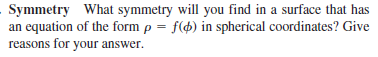### Create an Account

Home / Questions / Symmetry What symmetry will you find in a surface that has an equation of the form p = f(d...

# Symmetry What symmetry will you find in a surface that has an equation of the form p = f(d) in spherical coordinates? Give reasons for your answer.

Symmetry What symmetry will you find in a surface that has an equation of the form p = f(d) in spherical coordinates? Give reasons for your answer.

May 14 2021 View more View LessSubscribe To Get Solution